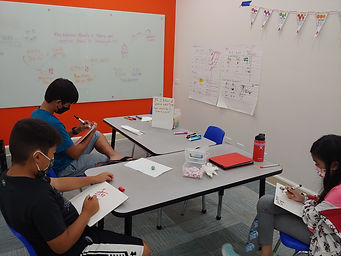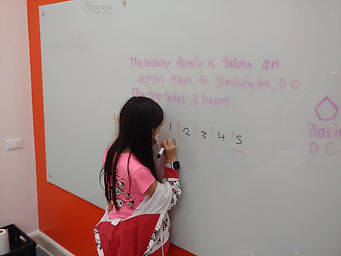## Ms. Mari

### Target 1​

###### Lesson Type:

Review

Data Probability

:

Ratio

Use ratio reasoning to calculate and compare unit price.

###### 1:

Find unit prices.

###### 2:

Use proportional reasoning to calculate the actual cost of individual items.

6th

###### Vocabulary:

RATIO, RATE, UNIT RATE, PROPORTION

Activities:

Determined unit rates and other equivalent rates to form proportions.### Home Exploration

###### Guiding Questions:## Absent Students:

Cameron

### Target 2

:

###### 1:

Use the graphic representation of a variable relationship to determine the proportions present.

###### 2:

Understand that the type of relationship present between two variables can easily be seen when graphed.

###### 3:

Use graphs, equations, and ordered pairs to examine the relationship between variables.

6th

###### Vocabulary:

PROPORTIONAL RELATIONSHIP

Activities:

Used equivalent rates to create a table of values.

Created a graph from the table of values.

Discussed characteristics of proportional relationships using the table and graph.### Home Exploration

###### Guiding Questions:### Target 3

:

###### Vocabulary:

Activities:### Home Exploration